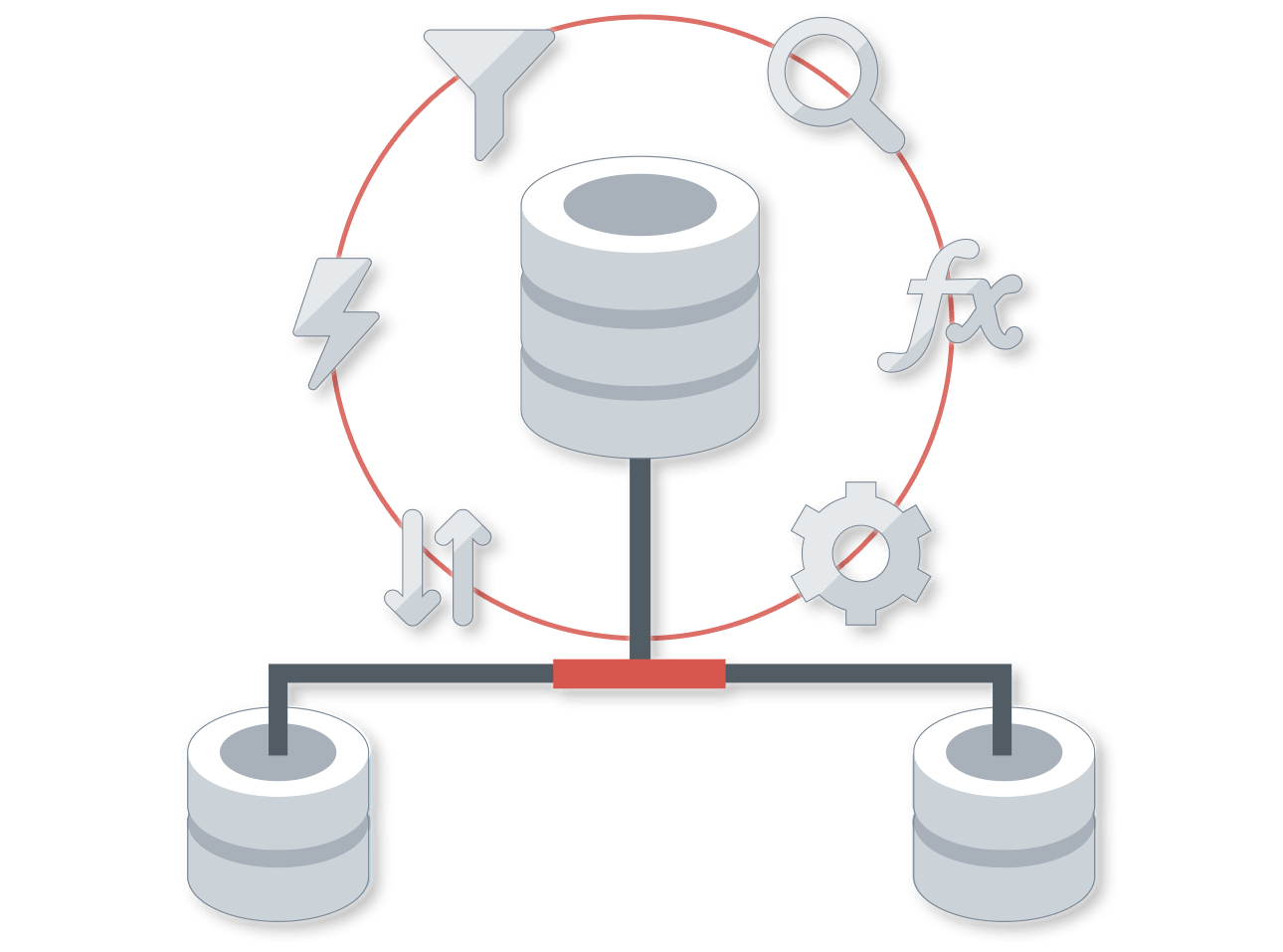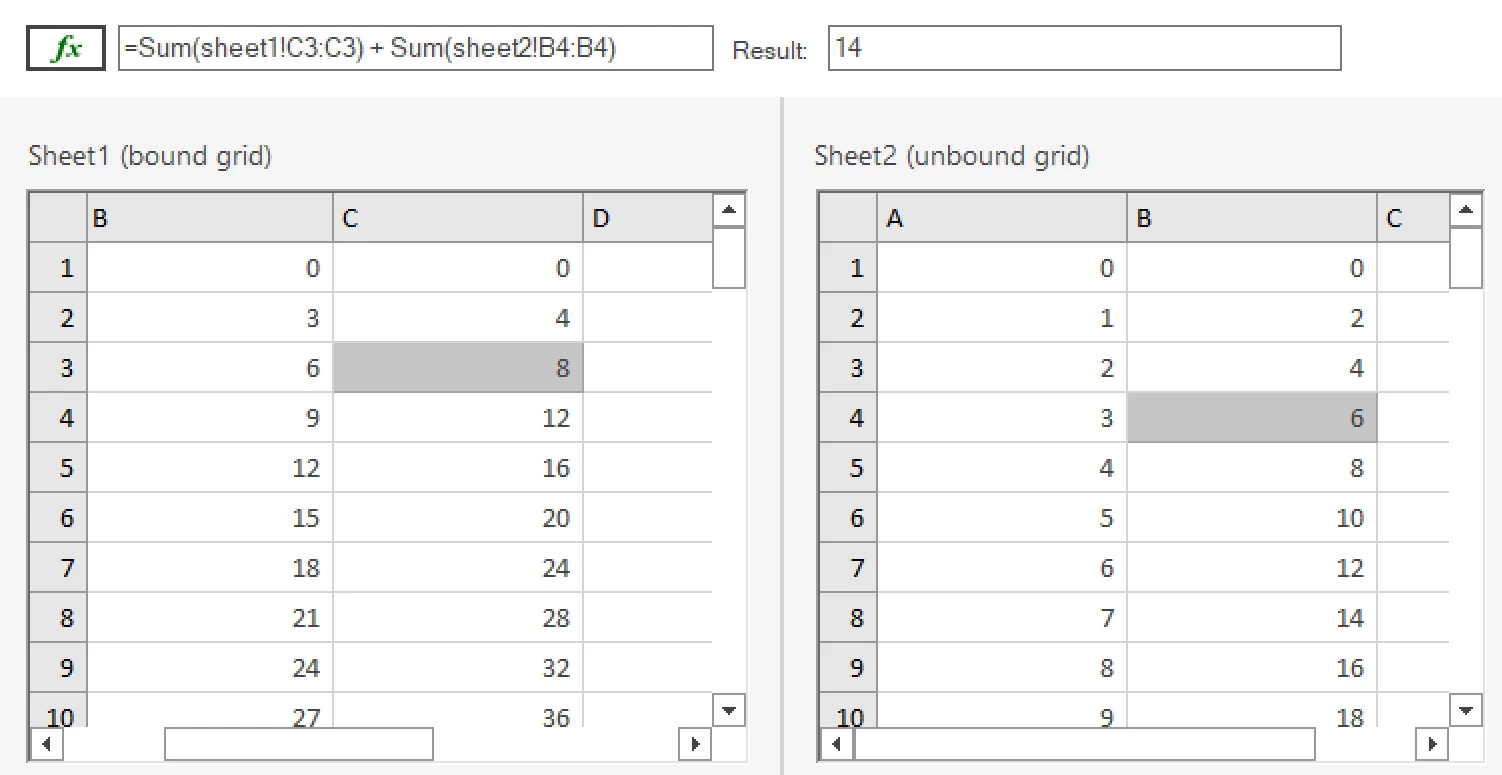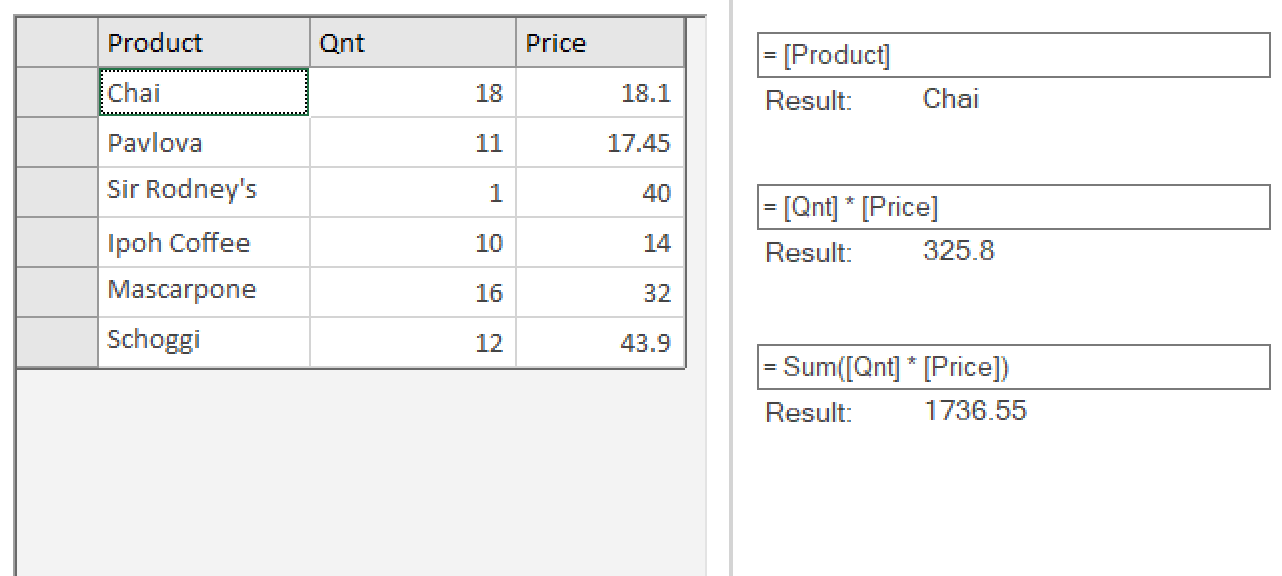# Easy .NET Expression Parser

Parse and evaluate expressions like Microsoft Excel and VBA with ComponentOne CalcEngine.

• Calculate algebraic and mathematical functions and formulas using variables at runtime
• Show computed values across datagrid cells just like Microsoft Excel
• Build a custom calculator for users to form equations against bound data fields

CalcEngine is available in WinForms, WPF and Data Services Edition

## Why Choose ComponentOne CalcEngine?Calculate sales projections, perform statistical calculations and find patterns in data, or show computed values in datagrid cells.Runtime Expression Parser

Save development time with our out-of-the-box .NET expression parser that evaluates the expressions automatically at runtime.Complete the Excel Experience

Combined with, ComponentOne CalcEngine provides the .NET formula bar functionality for any application.

## .NET Expression Parser Key Features

Function Support

CalcEngine supports an extensive, built-in function library including aggregate, date and time, logical, math and trigonometry, and text functions. It also supports custom functions and globalization.

Cross-Sheet References and Computation

CalcEngine can parse and compute algebraic expressions, mathematical functions, and formulas using variables and across multiple spreadsheets or controls.

Fast and Extensible Data Binding

CalcEngine can be bound to business objects, collection views, dynamic types, Entity Framework and DataTables. It can also be used to load Excel data into arbitrary data grid control, such as FlexGrid, and show computed values in cells.

## .NET Expression Parser EnginesMicrosoft Excel Formula Bar

Use CalcEngine to build a formula bar for parsing text expressions exactly like Microsoft Excel. For example, it can parse and compute algebraic expressions, mathematical functions, and formulas using variables across multiple spreadsheets or controls.Microsoft VBA Script Editor

Use CalcEngine to build a simple VBA-style calculator. For example, users can refer to field names using square brackets and perform mathematical functions against any data set to calculate expressions on the fly.

## Featured Blogs, Videos, and Other ResourcesIntroducing CalcEngine for .NET Creating an Excel-like Formula Bar in .NET WinFormsComponentOne DataEngine Deep-Dive FlexReport Designer SalesForce Integration FlexGrid for WinForms ApplicationsCalcEngine Overview Quick Start Licensing